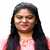# Calculators Created by Vidyashree VBMS College of Engineering (BMSCE), Bangalore
28
Formulas Created
51
Formulas Verified
20
Across Categories

## List of Calculators by Vidyashree V

Following is a combined list of all the calculators that have been created and verified by Vidyashree V. Vidyashree V has created 28 and verified 51 calculators across 20 different categories till date.
Verified Average Output Voltage of Three Phase 6 Pulse Diode Rectifier
Verified RMS Output Voltage of Three Phase 6 Pulse Diode Rectifier
BJT (2)
Verified BJT Turn OFF Time
Verified BJT Turn ON Time
5 More BJT Calculators
Verified Distance between Co-Channel Cells
15 More Cellular Concepts Calculators
Verified Number of Links in any Graph
14 More Circuit Graph Theory Calculators
Verified Current Division in Two Capacitors
Verified Voltage Division for Two Capacitors
15 More DC Circuits Calculators
Created Thermal Generation Rate
19 More Energy Band & Charge Carrier Calculators
Verified Bandwidth of FM wave by Carson Rule
Verified Bandwidth with respect to Modulation Index of FM
Verified Frequency Deviation
Verified Frequency Deviation provided Modulation Index
Verified Frequency Sensitivity
Verified Modulating Frequency
Verified Modulation Index of FM Wave
2 More Frequency Modulation Calculators
Verified Channel Reuse Ratio
Verified Coherence Bandwidth for Multipath Channel
Verified Maximum Doppler Shift
13 More Frequency Reuse Concept Calculators
Verified RMS Output Current of Single Phase Full Wave Midpoint Diode Rectifier with R Load
2 More Full Wave Calculators
Verified Bandwidth of Tuned Circuit
Verified Carrier Frequency
Verified Deviation Ratio
Verified Image Frequency
Verified Image Rejection Ratio
Verified Intermediate Frequency
Verified Modulation Index with respect to Power
Verified Quality Factor of Tuned Circuit
18 More Fundamentals of Analog Communications Calculators
Verified Average Voltage of Half Wave Thyristor Rectifier with RL Load
Verified Turn On Angle of Half Wave Rectifier
3 More Half Wave Calculators
Verified Average Load Current of Single Phase Half Wave Diode Rectifier with Resistive Load
Verified Average Output Current of Single Phase Half Wave Diode Rectifier with RE Load
Verified Average Output Voltage of Single Phase Half Wave Diode Rectifier with Resistive Load
Verified RMS Load Current of Single Phase Half Wave Diode Rectifier with RE Load
Verified RMS Load Current of Single Phase Half Wave Diode Rectifier with Resistive Load
Verified RMS Output Voltage of Single Phase Half Wave Diode Rectifier with Resistive Load
6 More Half Wave Calculators
Verified Average Output Voltage of Three Phase Half Wave Diode Rectifier with R Load in Phase Voltage Terms
3 More Half Wave Calculators
Created Level Crossing Rate
15 More Mobile Radio Propogation Calculators
MOSFET (1)
Verified Input Current Harmonic Factor
7 More MOSFET Calculators
Verified Schmitt Trigger Oscillator Hysteresis Constant
7 More Radio Frequency Range Calculators
Verified Emitter Voltage to Turn On UJT based Thyristor Firing Circuit
Verified Thyristor Firing Angle for RC Firing Circuit
Verified Time Period for UJT as Oscillator Thyristor Firing Circuit
7 More SCR Firing Circuit Calculators
Verified Average Load Voltage for Step down Chopper (Buck Converter)
Verified Average Load Voltage Step down Chopper (Buck Converter)
Verified Average Output Current for Step down Chopper (Buck Converter)
Verified RMS Load Voltage for Step down Chopper (Buck Converter)
4 More Step Up/Step Down Calculators
Created Beamwidth of Reflector
Created Current Maxima
Created Current Minima
Verified Cutoff Wavenumber in TM and TE Mode
Created Focal Length of Reflector
Created Gain of Parabolic Reflector Antenna
Created Minimum Distance from Antenna
Verified Parallel Waveguide Distance from Cutoff Wavenumber
Created Phase Constant in Telephone Cable
Created Polarization Mismatch Loss
Created Return Loss(dB)
Created Velocity Factor
Created Velocity of Propagation in Telephonic Cable
Created Voltage Maxima
Created Voltage Minima
Created Bandwidth of Antenna
Created Characteristic Impedance of Transmission Line
Created Current Standing Wave Ratio (CSWR)
Created Insertion Loss in Transmission Line
Created Length of Wound Conductor
Created Phase Velocity in Transmission Lines
Created Reflection Coefficient in Transmission Line
Created Relative Pitch of Wound Conductor
Created Resistance at Second Temperature
Created Return Loss by Means of VSWR
Created Standing Wave Ratio
Created Voltage Standing Wave Ratio (VSWR)
Created Wavelength of Line
2 More Transmission Line Characteristics CalculatorsLet Others Know